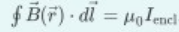# Problem: Learning goal: To understand Ampere's law and its application.Ampere's law is often writtenPart (a) The integral on the left is:A) the integral throught the chosen volumeB) the surface integral over the open surfaceC) the surface integral over the closed surface bounded by the loop.D) the line integral along the closed loop.E) the line integral from strat to finish. Part (b) The circle on the integral means the B(r) must be integratedA) Over a circle or a sphereB) along any closed path you choose.C) along the path of a closed physical conductor.D) over the surface bounded by the current-carrying wire.

###### FREE Expert Solution

Ampere's law:

$\overline{){\mathbf{\oint }}\stackrel{\mathbf{⇀}}{\mathbf{B}}{\mathbf{\left(}}\stackrel{\mathbf{⇀}}{\mathbf{r}}{\mathbf{\right)}}{\mathbf{·}}{\mathbf{d}}{\mathbf{l}}{\mathbf{=}}{{\mathbf{\mu }}}_{{\mathbf{0}}}{{\mathbf{i}}}_{\mathbf{e}\mathbf{n}\mathbf{c}\mathbf{l}}}$

Part (a)

The integral on the left is along the length.

91% (71 ratings)###### Problem Details

Learning goal: To understand Ampere's law and its application.

Ampere's law is often writtenPart (a) The integral on the left is:

A) the integral throught the chosen volume
B) the surface integral over the open surface
C) the surface integral over the closed surface bounded by the loop.
D) the line integral along the closed loop.
E) the line integral from strat to finish.

Part (b) The circle on the integral means the B(r) must be integrated

A) Over a circle or a sphere
B) along any closed path you choose.
C) along the path of a closed physical conductor.
D) over the surface bounded by the current-carrying wire.